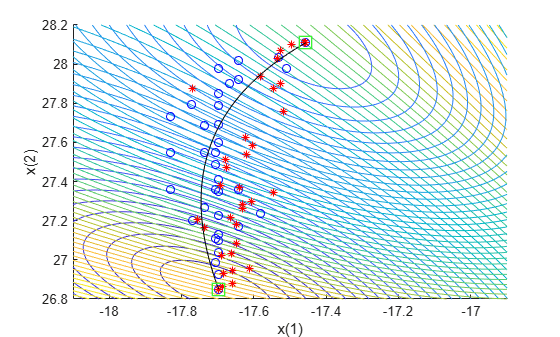# Compare `paretosearch` and `gamultiobj`

This example shows how to create a set of points on the Pareto front using both `paretosearch` and `gamultiobj`. The objective function has two objectives and a two-dimensional control variable `x`. The objective function `mymulti3` is available in your MATLAB® session when you click the button to edit or try this example. Alternatively, copy the `mymulti3` code to your session. For speed of calculation, the function is vectorized.

`type mymulti3`
```function f = mymulti3(x) % f(:,1) = x(:,1).^4 + x(:,2).^4 + x(:,1).*x(:,2) - (x(:,1).^2).*(x(:,2).^2) - 9*x(:,1).^2; f(:,2) = x(:,2).^4 + x(:,1).^4 + x(:,1).*x(:,2) - (x(:,1).^2).*(x(:,2).^2) + 3*x(:,2).^3; ```

### Basic Example and Plots

Find Pareto sets for the objective functions using `paretosearch` and `gamultiobj`. Set the `UseVectorized` option to `true` for added speed. Include a plot function to visualize the Pareto set.

```rng default nvars = 2; opts = optimoptions(@gamultiobj,'UseVectorized',true,'PlotFcn','gaplotpareto'); [xga,fvalga,~,gaoutput] = gamultiobj(@(x)mymulti3(x),nvars,[],[],[],[],[],[],[],opts);```
```Optimization terminated: average change in the spread of Pareto solutions less than options.FunctionTolerance. ```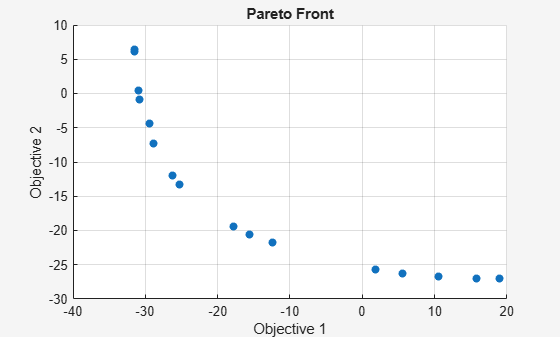```optsp = optimoptions('paretosearch','UseVectorized',true,'PlotFcn',{'psplotparetof' 'psplotparetox'}); [xp,fvalp,~,psoutput] = paretosearch(@(x)mymulti3(x),nvars,[],[],[],[],[],[],[],optsp);```
```Pareto set found that satisfies the constraints. Optimization completed because the relative change in the volume of the Pareto set is less than 'options.ParetoSetChangeTolerance' and constraints are satisfied to within 'options.ConstraintTolerance'. ```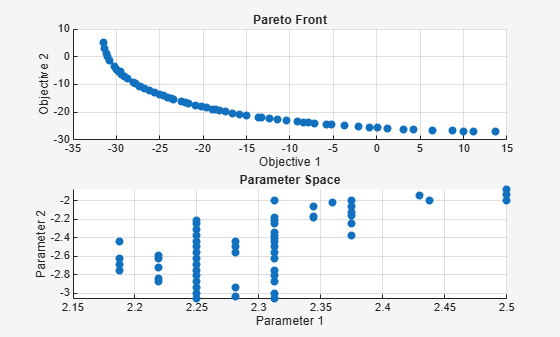Compute theoretically exact points on the Pareto front by using `mymulti4`. The `mymulti4` function is available in your MATLAB session when you click the button to edit or try this example.

`type mymulti4`
```function mout = mymulti4(x) % gg = [4*x(1)^3+x(2)-2*x(1)*(x(2)^2) - 18*x(1); x(1)+4*x(2)^3-2*(x(1)^2)*x(2)]; gf = gg + [18*x(1);9*x(2)^2]; mout = gf(1)*gg(2) - gf(2)*gg(1); ```

The `mymulti4` function evaluates the gradients of the two objective functions. Next, for a range of values of `x(2)`, use `fzero` to locate the point where the gradients are exactly parallel, which is where the output `mout` = 0.

```a = [fzero(@(t)mymulti4([t,-3.15]),[2,3]),-3.15]; for jj = linspace(-3.125,-1.89,50) a = [a;[fzero(@(t)mymulti4([t,jj]),[2,3]),jj]]; end figure plot(fvalp(:,1),fvalp(:,2),'bo'); hold on fs = mymulti3(a); plot(fvalga(:,1),fvalga(:,2),'r*'); plot(fs(:,1),fs(:,2),'k.') legend('Paretosearch','Gamultiobj','True') xlabel('Objective 1') ylabel('Objective 2') hold off```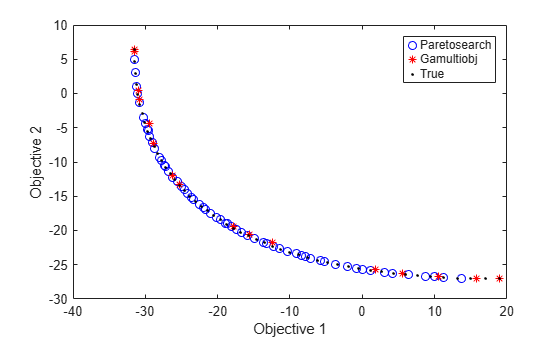`gamultiobj` finds points with a slightly wider spread in objective function space. Plot the solutions in decision variable space, along with the theoretical optimal Pareto curve and a contour plot of the two objective functions.

```[x,y] = meshgrid(1.9:.01:3.1,-3.2:.01:-1.8); mydata = mymulti3([x(:),y(:)]); myff = sqrt(mydata(:,1) + 39);% Spaces the contours better mygg = sqrt(mydata(:,2) + 28);% Spaces the contours better myff = reshape(myff,size(x)); mygg = reshape(mygg,size(x)); figure; hold on contour(x,y,mygg,50) contour(x,y,myff,50) plot(xp(:,1),xp(:,2),'bo') plot(xga(:,1),xga(:,2),'r*') plot(a(:,1),a(:,2),'-k') xlabel('x(1)') ylabel('x(2)') hold off```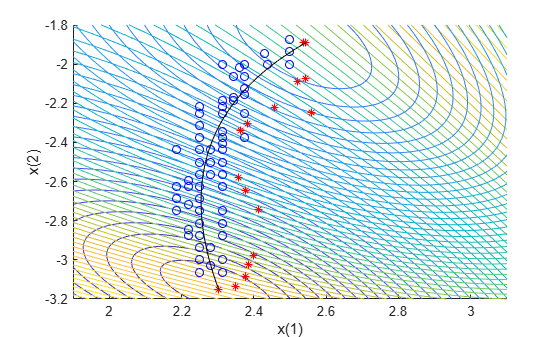Unlike the `paretosearch` solution, the `gamultiobj` solution has points at the extreme ends of the range in objective function space. However, the `paretosearch` solution has more points that are closer to the true solution in both objective function space and decision variable space. The number of points on the Pareto front is different for each solver when you use the default options.

### Shifted Problem

What happens if the solution to your problem has control variables that are large? Examine this case by shifting the problem variables. For an unconstrained problem, `gamultiobj` can fail, while `paretosearch` is more robust to such shifts.

For easier comparison, specify 35 points on the Pareto front for each solver.

```shift = [20,-30]; fun = @(x)mymulti3(x+shift); opts.PopulationSize = 100; % opts.ParetoFraction = 35 [xgash,fvalgash,~,gashoutput] = gamultiobj(fun,nvars,[],[],[],[],[],[],opts);```
```Optimization terminated: average change in the spread of Pareto solutions less than options.FunctionTolerance. ```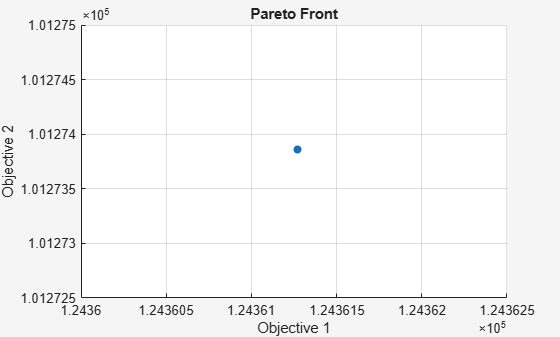`gamultiobj` fails to find a useful Pareto set.

```optsp.PlotFcn = []; optsp.ParetoSetSize = 35; [xpsh,fvalpsh,~,pshoutput] = paretosearch(fun,nvars,[],[],[],[],[],[],[],optsp);```
```Pareto set found that satisfies the constraints. Optimization completed because the relative change in the volume of the Pareto set is less than 'options.ParetoSetChangeTolerance' and constraints are satisfied to within 'options.ConstraintTolerance'. ```
```figure plot(fvalpsh(:,1),fvalpsh(:,2),'bo'); hold on plot(fs(:,1),fs(:,2),'k.') legend('Paretosearch','True') xlabel('Objective 1') ylabel('Objective 2') hold off```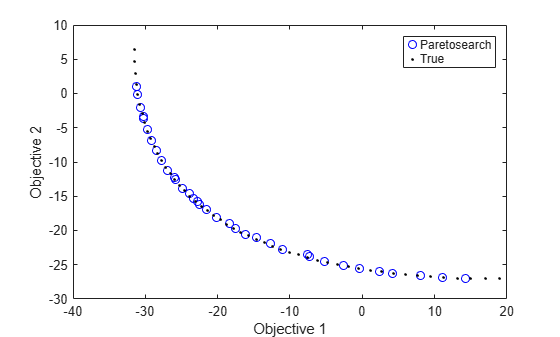`paretosearch` finds solution points spread evenly over nearly the entire possible range.

Adding bounds, even fairly loose ones, helps both `gamultiobj` and `paretosearch` to find appropriate solutions. Set lower bounds of `-50` in each component, and upper bounds of `50`.

```opts.PlotFcn = []; optsp.PlotFcn = []; lb = [-50,-50]; ub = -lb; [xgash,fvalgash,~,gashoutput] = gamultiobj(fun,nvars,[],[],[],[],lb,ub,opts);```
```Optimization terminated: average change in the spread of Pareto solutions less than options.FunctionTolerance. ```
`[xpsh2,fvalpsh2,~,pshoutput2] = paretosearch(fun,nvars,[],[],[],[],lb,ub,[],optsp);`
```Pareto set found that satisfies the constraints. Optimization completed because the relative change in the volume of the Pareto set is less than 'options.ParetoSetChangeTolerance' and constraints are satisfied to within 'options.ConstraintTolerance'. ```
```figure plot(fvalpsh2(:,1),fvalpsh2(:,2),'bo'); hold on plot(fvalgash(:,1),fvalgash(:,2),'r*'); plot(fs(:,1),fs(:,2),'k.') legend('Paretosearch','Gamultiobj','True') xlabel('Objective 1') ylabel('Objective 2') hold off```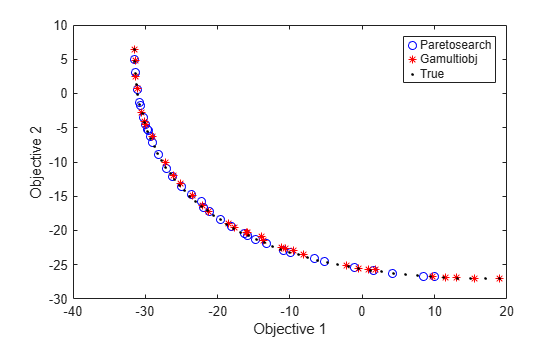In this case, both solvers find good solutions.

### Start `paretosearch` from `gamultiobj` Solution

Obtain a similar range of solutions from the solvers by starting `paretosearch` from the `gamultiobj` solution.

```optsp.InitialPoints = xgash; [xpsh3,fvalpsh3,~,pshoutput3] = paretosearch(fun,nvars,[],[],[],[],lb,ub,[],optsp);```
```Pareto set found that satisfies the constraints. Optimization completed because the relative change in the volume of the Pareto set is less than 'options.ParetoSetChangeTolerance' and constraints are satisfied to within 'options.ConstraintTolerance'. ```
```figure plot(fvalpsh3(:,1),fvalpsh3(:,2),'bo'); hold on plot(fvalgash(:,1),fvalgash(:,2),'r*'); plot(fs(:,1),fs(:,2),'k.') legend('Paretosearch','Gamultiobj','True') xlabel('Objective 1') ylabel('Objective 2') hold off```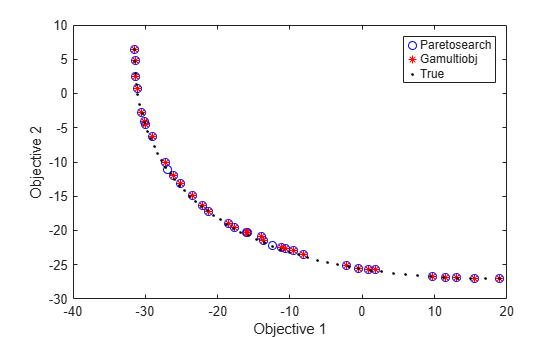Now the `paretosearch` solution is similar to the `gamultiobj` solution, although some of the solution points differ.

### Start from Single-Objective Solutions

Another way of obtaining a good solution is to start from the points that minimize each objective function separately.

From the multiobjective function, create a single-objective function that chooses each objective in turn. Use the shifted function from the previous section. Because you are giving good start points to the solvers, you do not need to specify bounds.

```nobj = 2; % Number of objectives x0 = -shift; % Initial point for single-objective minimization uncmin = cell(nobj,1); % Cell array to hold the single-objective minima allfuns = zeros(nobj,2); % Hold the objective function values eflag = zeros(nobj,1); fopts = optimoptions('patternsearch','Display','off'); % Use an appropriate solver here for i = 1:nobj indi = zeros(nobj,1); % Choose the objective to minimize indi(i) = 1; funi = @(x)dot(fun(x),indi); [uncmin{i},~,eflag(i)] = patternsearch(funi,x0,[],[],[],[],[],[],[],fopts); % Minimize objective i allfuns(i,:) = fun(uncmin{i}); end uncmin = cell2mat(uncmin); % Matrix of start points```

Start `paretosearch` from the single-objective minimum points and note that it has a full range in its solutions. `paretosearch` adds random initial points to the supplied ones in order to have a population of at least `options.ParetoSetSize` individuals. Similarly, `gamultiobj` adds random points to the supplied ones to obtain a population of at least `(options.PopulationSize)*(options.ParetoFraction)` individuals.

```optsp = optimoptions(optsp,'InitialPoints',uncmin); [xpinit,fvalpinit,~,outputpinit] = paretosearch(fun,nvars,[],[],[],[],[],[],[],optsp);```
```Pareto set found that satisfies the constraints. Optimization completed because the relative change in the volume of the Pareto set is less than 'options.ParetoSetChangeTolerance' and constraints are satisfied to within 'options.ConstraintTolerance'. ```

Now solve the problem using `gamultiobj` starting from the initial points.

```opts = optimoptions(opts,'InitialPopulationMatrix',uncmin); [xgash2,fvalgash2,~,gashoutput2] = gamultiobj(fun,nvars,[],[],[],[],[],[],opts);```
```Optimization terminated: average change in the spread of Pareto solutions less than options.FunctionTolerance. ```
```figure plot(fvalpinit(:,1),fvalpinit(:,2),'bo'); hold on plot(fvalgash2(:,1),fvalgash2(:,2),'r*'); plot(fs(:,1),fs(:,2),'k.') plot(allfuns(:,1),allfuns(:,2),'gs','MarkerSize',12) legend('Paretosearch','Gamultiobj','True','Start Points') xlabel('Objective 1') ylabel('Objective 2') hold off```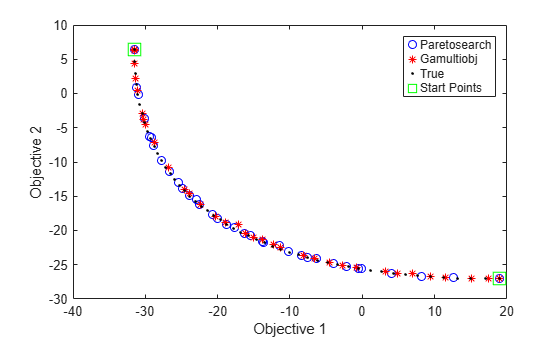Both solvers fill in the Pareto front between the extreme points, with reasonably accurate and well-spaced solutions.

View the final points in decision variable space.

```figure; hold on xx = x - shift(1); yy = y - shift(2); contour(xx,yy,mygg,50) contour(xx,yy,myff,50) plot(xpinit(:,1),xpinit(:,2),'bo') plot(xgash2(:,1),xgash2(:,2),'r*') ashift = a - shift; plot(ashift(:,1),ashift(:,2),'-k') plot(uncmin(:,1),uncmin(:,2),'gs','MarkerSize',12); xlabel('x(1)') ylabel('x(2)') hold off```